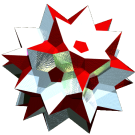Acronym sladit Name small lanceal ditriacontahedron,strombic hexecontahedron,deltoidal hexecontahedron,tetragonal hexecontahedron© Inradius sqrt[(97+43 sqrt(5))/82] = 1.534764 Dihedral angles at long edge:   arccos[-(19+8 sqrt(5))/41] = 154.121363° at short edge:   arccos[-(19+8 sqrt(5))/41] = 154.121363° Dual srid ExternallinksOnly the lacings c and d survive as true edges. Those are the long resp. short ones. For their ratio one furthermore derives c/d = (7+sqrt(5))/6 = 1.539345. All other distances given below qualify as pseude edges only. Esp. x is nothing but the girding diagonal of the kites, and e is just the axial diagonal of the kites.

Incidence matrix according to Dynkin symbol

m3o5m =
aoo3oxo5oob&#z(c,d,e)   → height = 0
a = (3+sqrt(5))/3 = 1.745356
b = (8+2 sqrt(5))/11 = 1.133831
c = lacing(1,2) = sqrt[5+sqrt(5)]/3 = 0.896665
d = lacing(2,3) = sqrt[50-4 sqrt(5)]/11 = 0.582498
e = lacing(1,3) = sqrt[626+250 sqrt(5)]/33 = 1.043154

o..3o..5o..         | 12  *  * |  4  0 |  4
.o.3.o.5.o.         |  * 30  * |  2  2 |  4
..o3..o5..o         |  *  * 20 |  0  3 |  3
--------------------+----------+-------+---
oo.3oo.5oo.&#c      |  1  1  0 | 60  * |  2  c
.oo3.oo5.oo&#d      |  0  1  1 |  * 60 |  2  d
--------------------+----------+-------+---
... oxo ...&#(c,d)t |  1  2  1 |  2  2 | 60